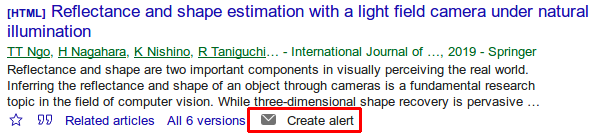TL;DR:Get direct Create alert links on Google Scholar listings, even for uncited papers, by using this script in Greasemonkey (Firefox) or this script in Tampermonkey (Chrome).

Matlab decomposition objects in parfor

In Matlab 2017b Mathworks introduced the decomposition class, which precomputes a decomposition of a linear system:

>> A = rand(10000, 10000);
>> b = rand(10000, 1);
>> tic; x = A \ b; toc
Elapsed time is 5.049433 seconds.

>> tic; dA = decomposition(A); toc
Elapsed time is 4.916148 seconds.

>> tic; x2 = dA \ b; toc
Elapsed time is 0.039961 seconds.

>> nnz(x ~= x2)
ans = 0

As can be seen, once the decomposition object is created, this allows solving the system at least two orders of magnitude faster with the exact same results.

The decomposition class is as smart as mldivide (backslash operator for solving Ax = b) and mrdivide (forward slash operator for solving xA = b) and follows the same logic as can be seen in the flow charts for dense or sparse matrices. That means it recognizes special kinds of system matrices and performs a suitable decomposition that exploits the matrix structure.